xFibrationEncyclopedia
In topology
Topology
Topology is a major area of mathematics concerned with properties that are preserved under continuous deformations of objects, such as deformations that involve stretching, but no tearing or gluing...

, a branch of mathematics, a fibration is a generalization of the notion of a fiber bundle
Fiber bundle
In mathematics, and particularly topology, a fiber bundle is intuitively a space which locally "looks" like a certain product space, but globally may have a different topological structure...

. A fiber bundle makes precise the idea of one topological space
Topological space
Topological spaces are mathematical structures that allow the formal definition of concepts such as convergence, connectedness, and continuity. They appear in virtually every branch of modern mathematics and are a central unifying notion...

(called a fiber) being "parameterized" by another topological space (called a base). A fibration is like a fiber bundle, except that the fibers need not be the same space, rather they are just homotopy equivalent. Fibrations do not necessarily have the local Cartesian product
Cartesian product
In mathematics, a Cartesian product is a construction to build a new set out of a number of given sets. Each member of the Cartesian product corresponds to the selection of one element each in every one of those sets...

structure that defines the more restricted fiber bundle case, but something weaker that still allows "sideways" movement from fiber to fiber. Fiber bundles have a particularly simple homotopy theory that allows topological information about the bundle to be inferred from information about one or both of these constituent spaces. A fibration satisfies an additional condition (the homotopy lifting property
Homotopy lifting property
In mathematics, in particular in homotopy theory within algebraic topology, the homotopy lifting property is a technical condition on a continuous function from a topological space E to another one, B...

) guaranteeing that it will behave like a fiber bundle from the point of view of homotopy theory.

## Formal definition

A fibration is a continuous mapping  satisfying the homotopy lifting property
Homotopy lifting property
In mathematics, in particular in homotopy theory within algebraic topology, the homotopy lifting property is a technical condition on a continuous function from a topological space E to another one, B...

with respect to any space. Fiber bundle
Fiber bundle
In mathematics, and particularly topology, a fiber bundle is intuitively a space which locally "looks" like a certain product space, but globally may have a different topological structure...

s (over paracompact bases) constitute important examples. In homotopy theory any mapping is 'as good as' a fibration — i.e. any map can be decomposed as a homotopy equivalence into a "mapping path space" followed by a fibration. (See homotopy fiber
Homotopy fiber
In mathematics, especially homotopy theory, the homotopy fiber is part of a construction that associates a fibration to an arbitrary continuous function of topological spaces f \colon A \to B....

.)

The fibers are by definition the subspaces of E that are the inverse images of points b of B. If the base space B is path connected, it is a consequence of the definition that the fibers of two different points b1 and b2 in B are homotopy equivalent. Therefore one usually speaks of "the fiber" F.

## Serre fibrations

A continuous mapping with the homotopy lifting property for CW complex
CW complex
In topology, a CW complex is a type of topological space introduced by J. H. C. Whitehead to meet the needs of homotopy theory. This class of spaces is broader and has some better categorical properties than simplicial complexes, but still retains a combinatorial naturethat allows for...

es (or equivalently, just cubes In) is called a Serre fibration, in honor of the part played by the concept in the thesis of Jean-Pierre Serre
Jean-Pierre Serre
Jean-Pierre Serre is a French mathematician. He has made contributions in the fields of algebraic geometry, number theory, and topology.-Early years:...

. This thesis firmly established in algebraic topology
Algebraic topology
Algebraic topology is a branch of mathematics which uses tools from abstract algebra to study topological spaces. The basic goal is to find algebraic invariants that classify topological spaces up to homeomorphism, though usually most classify up to homotopy equivalence.Although algebraic topology...

the use of spectral sequence
Spectral sequence
In homological algebra and algebraic topology, a spectral sequence is a means of computing homology groups by taking successive approximations...

s, and clearly separated the notions of fiber bundles and fibrations from the notion of sheaf
Sheaf (mathematics)
In mathematics, a sheaf is a tool for systematically tracking locally defined data attached to the open sets of a topological space. The data can be restricted to smaller open sets, and the data assigned to an open set is equivalent to all collections of compatible data assigned to collections of...

(both concepts together having been implicit in the pioneer treatment of Jean Leray
Jean Leray
Jean Leray was a French mathematician, who worked on both partial differential equations and algebraic topology....

). Because a sheaf (thought of as an étalé space) can be considered a local homeomorphism
Local homeomorphism
In mathematics, more specifically topology, a local homeomorphism is intuitively a function, f, between topological spaces that preserves local structure. Equivalently, one can cover the domain of this function by open sets, such that f restricted to each such open set is a homeomorphism onto its...

, the notions were closely interlinked at the time. One of the main desirable properties of the Serre spectral sequence
Serre spectral sequence
In mathematics, the Serre spectral sequence is an important tool in algebraic topology...

is to account for the action of the fundamental group
Fundamental group
In mathematics, more specifically algebraic topology, the fundamental group is a group associated to any given pointed topological space that provides a way of determining when two paths, starting and ending at a fixed base point, can be continuously deformed into each other...

of the base B on the homology of the total space E.

## Examples

In the following examples a fibration is denoted
FEB,

where the first map is the inclusion of "the" fiber F into the total space E and the second map is the fibration onto the basis B. This is also referred to as a fibration sequence.
• The projection map from a product space is very easily seen to be a fibration.
• Fiber bundle
Fiber bundle
In mathematics, and particularly topology, a fiber bundle is intuitively a space which locally "looks" like a certain product space, but globally may have a different topological structure...

s have local trivializations such Cartesian product structures exist locally on B, and this is usually enough to show that a fiber bundle is a fibration. More precisely, if there are local trivializations over a "numerable open cover" of B , the bundle is a fibration. Any open cover of a paracompact space is numerable. For example, any open cover of a metric space has a locally finite refinement, so any bundle over such a space is a fibration. The local triviality also implies the existence of a well-defined
Well-defined
In mathematics, well-definition is a mathematical or logical definition of a certain concept or object which uses a set of base axioms in an entirely unambiguous way and satisfies the properties it is required to satisfy. Usually definitions are stated unambiguously, and it is clear they satisfy...

fiber (up to
Up to
In mathematics, the phrase "up to x" means "disregarding a possible difference in  x".For instance, when calculating an indefinite integral, one could say that the solution is f "up to addition by a constant," meaning it differs from f, if at all, only by some constant.It indicates that...

homeomorphism
Homeomorphism
In the mathematical field of topology, a homeomorphism or topological isomorphism or bicontinuous function is a continuous function between topological spaces that has a continuous inverse function. Homeomorphisms are the isomorphisms in the category of topological spaces—that is, they are...

), at least on each connected component
Connected space
In topology and related branches of mathematics, a connected space is a topological space that cannot be represented as the union of two or more disjoint nonempty open subsets. Connectedness is one of the principal topological properties that is used to distinguish topological spaces...

of B.
• The Hopf fibration S1S3S2 was historically one of the earliest non-trivial examples of a fibration.
• The Serre fibration SO(2) → SO(3) → S2 comes from the action of the rotation group
Rotation group
In mechanics and geometry, the rotation group is the group of all rotations about the origin of three-dimensional Euclidean space R3 under the operation of composition. By definition, a rotation about the origin is a linear transformation that preserves length of vectors and preserves orientation ...

SO(3) on the 2-sphere
Sphere
A sphere is a perfectly round geometrical object in three-dimensional space, such as the shape of a round ball. Like a circle in two dimensions, a perfect sphere is completely symmetrical around its center, with all points on the surface lying the same distance r from the center point...

S2.
• Over complex projective space
Complex projective space
In mathematics, complex projective space is the projective space with respect to the field of complex numbers. By analogy, whereas the points of a real projective space label the lines through the origin of a real Euclidean space, the points of a complex projective space label the complex lines...

, there is a fibration S1S2n+1CPn
Complex projective space
In mathematics, complex projective space is the projective space with respect to the field of complex numbers. By analogy, whereas the points of a real projective space label the lines through the origin of a real Euclidean space, the points of a complex projective space label the complex lines...

.

### Euler characteristic

The Euler characteristic
Euler characteristic
In mathematics, and more specifically in algebraic topology and polyhedral combinatorics, the Euler characteristic is a topological invariant, a number that describes a topological space's shape or structure regardless of the way it is bent...

is multiplicative for fibrations with certain conditions.

If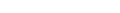is a fibration with fiber F, with the base B path-connected, and the fibration is orientable over a field K, then the Euler characteristic with coefficients in the field K satisfies the product property: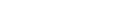This includes product spaces and covering spaces as special cases,
and can be proven by the Serre spectral sequence
Serre spectral sequence
In mathematics, the Serre spectral sequence is an important tool in algebraic topology...

on homology of a fibration.

For fiber bundles, this can also be understood in terms of a transfer map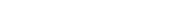– note that this is a lifting and goes "the wrong way" – whose composition with the projection map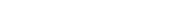is multiplication by the Euler class of the fiber: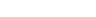## Fibrations in closed model categories

Fibrations of topological spaces fit into a more general framework, the so-called closed model categories. In such categories, there are distinguished classes of morphisms, the so-called fibrations, cofibration
Cofibration
In mathematics, in particular homotopy theory, a continuous mappingi\colon A \to X,where A and X are topological spaces, is a cofibration if it satisfies the homotopy extension property with respect to all spaces Y. The name is because the dual condition, the homotopy lifting property, defines...

s
and weak equivalence
Weak equivalence
-Mathematics:In mathematics, a weak equivalence is a notion from homotopy theory which in some sense identifies objects that have the same basic "shape"...

s
. Certain axiom
Axiom
In traditional logic, an axiom or postulate is a proposition that is not proven or demonstrated but considered either to be self-evident or to define and delimit the realm of analysis. In other words, an axiom is a logical statement that is assumed to be true...

s, such as stability of fibrations under composition and pullbacks
Pullback (category theory)
In category theory, a branch of mathematics, a pullback is the limit of a diagram consisting of two morphisms f : X → Z and g : Y → Z with a common codomain; it is the limit of the cospan X \rightarrow Z \leftarrow Y...

, factorization of every morphism into the composition of an acyclic cofibration followed by a fibration or a cofibration followed by an acyclic fibration, where the word "acyclic" indicates that the corresponding arrow is also a weak equivalence, and other requirements are set up to allow the abstract treatment of homotopy theory. (In the original treatment, due to Daniel Quillen, the word "trivial" was used instead of "acyclic.")

It can be shown that the category of topological spaces is in fact a model category, where (abstract) fibrations are just the Serre fibrations introduced above and weak equivalences are weak homotopy equivalences.
The source of this article is wikipedia, the free encyclopedia.  The text of this article is licensed under the GFDL.